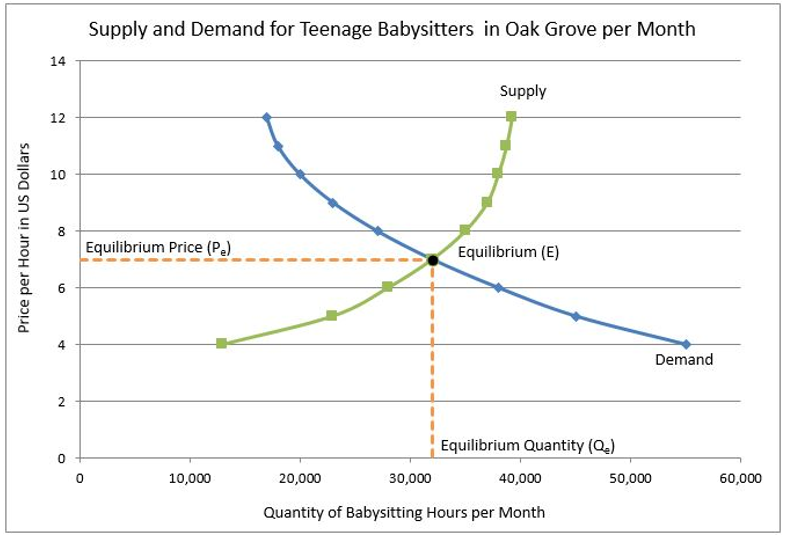# Equilibrium Price

## Definition of Equilibrium Price:

The equilibrium price is the resulting price of a good or service when the quantity demanded equals the quantity supplied. It is the price where the supply and demand curves intersect.

## Detailed Explanation:

A supply and demand graph illustrates how a market arrives at an equilibrium price. The graph below is for babysitters in the Oak Grove community.The equilibrium price is the price where the quantity demanded by consumers equals the quantity supplied by producers. The equilibrium quantity is the quantity of a good or service bought and produced at the equilibrium price. Notice that at a price of \$7 per hour, consumers demand 32,000 babysitting hours and sitters are willing to provide 32,000 hours of babysitting. Seven dollars is the equilibrium price (Pe) and 32,000 babysitting hours is the equilibrium quantity (Qe). Equilibrium (E) occurs when the quantity supplied equals the quantity demanded. This is where the supply and demand curves intersect. Let us see how market forces push a product’s price and the quantity produced to the equilibrium levels.

A shortage results when the quantity supplied is less than the quantity demanded at a given price. Consumers will bid prices higher to provide suppliers a greater incentive to produce more. Shortages exist at all prices below the equilibrium price. For example, at a price of \$6 per hour, parents would demand 38,000 babysitting hours, but sitters would only be enticed to provide 28,000 hours. In such a case, there would be a shortage of 10,000 hours. Some parents would struggle to find a babysitter and bid the price up to find someone to sit for their child. Eventually the price would move to \$7 per hour.

A surplus occurs when the quantity demanded is less than the quantity supplied at a given price. Market forces will push the price down to equilibrium. Returning to our babysitting example, sitters would be willing to babysit 35,000 hours if the price was \$8 per hour, but parents would only be willing to purchase 27,000 hours, resulting in a surplus of 8,000 hours. Some babysitters would cut their rate to get more business, and others might leave the business.

Market forces will push the market price and quantity supplied to equilibrium. Constant adjustments are made in the marketplace. A change in the demand or supply of a good or service will force an adjustment in the equilibrium price. An increase in the demand or an decrease in the supply result in an increase in the equilibrium price. Conversely if the demand decreases, or the supply increases, the equilibrium price will decrease.

## Dig Deeper With These Free Lessons:

Supply and Demand - Consumers and Producers Reach Agreement
Price Elasticity of Demand - How Much of a Price Change Would You Tolerate
Price Elasticity of Supply - How Does a Producer Respond to a Price Change
Supply and Demand - The Costs and Benefits of Price Controls
Supply and Demand - The Costs and Benefits of Restricting Supply
Supply and Demand - Understand The Dynamics of The Stock Market
Supply and Demand - Who Pays an Excise Tax